# Construction: Dividing a Line Segment into N Equal Parts

Compass and straight edge constructions are of interest to mathematicians, not only in the field of geometry, but also in algebra. Ancient Greek mathematicians were interested in the problem of "trisecting an angle" (splitting an arbitrary angle into three equal parts) using only a straight edge and compass. It turns out that some, but not all angles can be trisected, but no-one managed to prove that some angles could not be trisected using a straight edge and compass until the 19th Century!

However, it is possible to trisect a line segment, or divide it into any number of equal parts, using only a straight edge and compass.

For this construction, you will need a straight edge (ruler - but you won't be measuring anything), pair of compasses, a pencil and paper. I have drawn the pictures using the robocompass app. It's fun to play with, and you can use it to do all sorts of geometric constructions. There's a little bit of coding to learn, but a list of instructions is provided. Once you've written your little program, you can invite a few friends over, settle down in some comfy chairs, bring out the popcorn, sit back, relax and enjoy the show. Be warned that, when you are using it to draw arcs, the robocompass compass point may appear to be a little away from the centre, but the drawing is actually accurate.

## The Construction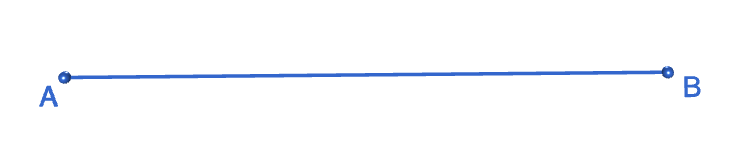Step 1: Start with the line segment $AB$ that you want to cut into parts.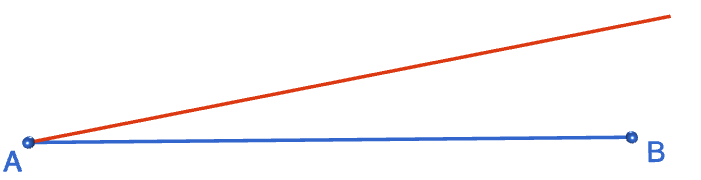Step 2: Draw a second line segment, starting at point $A$ that is at an angle to $AB$ and about the same length as $AB$.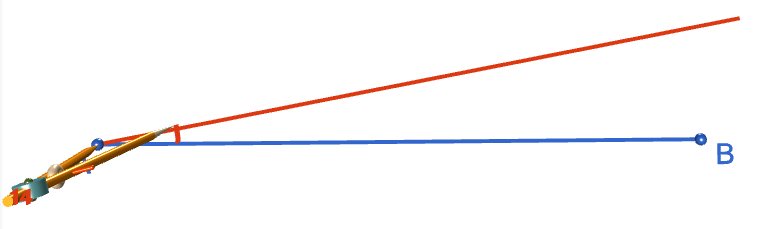Step 3: Decide how many parts you want to divide your line segment into. I chose $8$, so I opened my compasses to slightly less than one eighth of the length of the new line I drew.

Starting at point $A$, I drew an arc of this radius across my new line. I then moved the tip of the compasses to the point of intersection of the arc with the new line and drew a second arc of the same radius across the line. Keep repeating this process until you have the desired number of arcs (8 in my case). Label the point where the last one cuts the new line with a $C$. Make sure each arc is centred at the preceding point of intersection.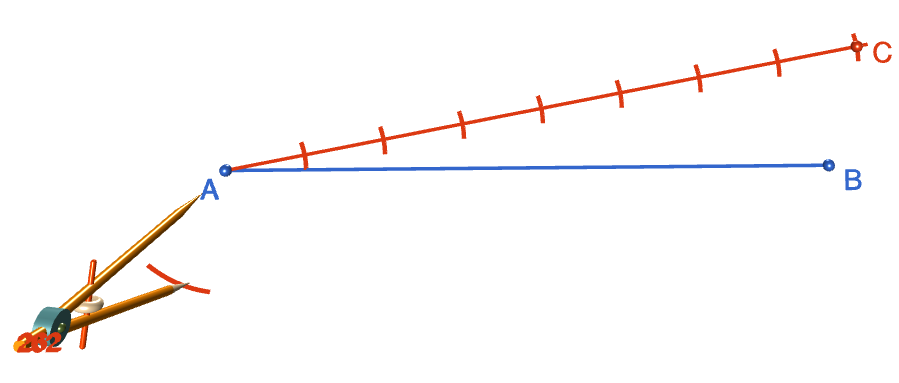Step 4: Set the radius of the compasses to the distance between $C$ and $B$. Move the tip of the compasses to point $A$, and draw an arc of this radius just below $A$. The picture shows the tip of the compasses a little way away from point $A$, but please make sure you centre your compasses at $A$.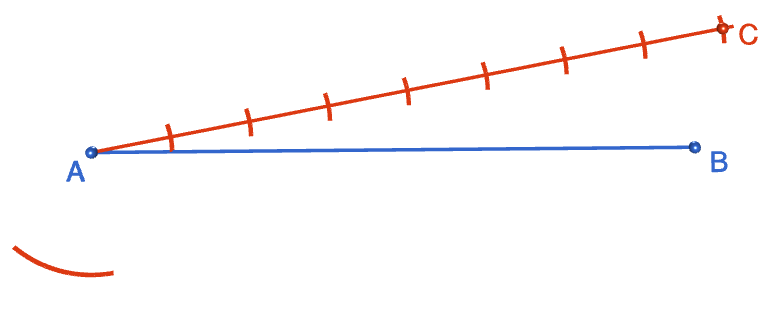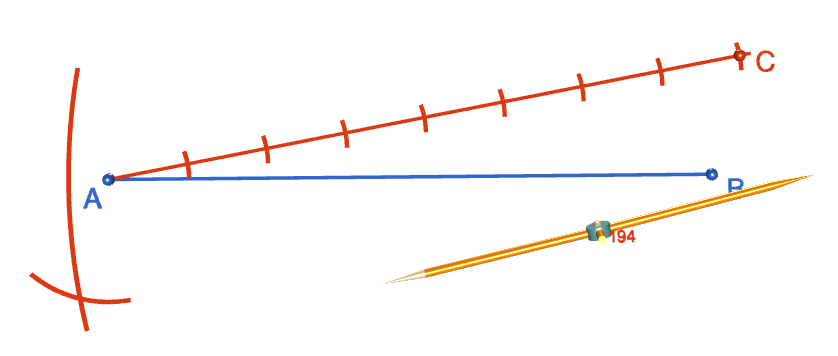Step 5: Open out the compasses to a radius that matches the length of $AC$. Move the tip of the compasses to the point $B$ and draw an arc that crosses the arc drawn in the preceding step. Label the point of intersection of the two arcs with a $D$.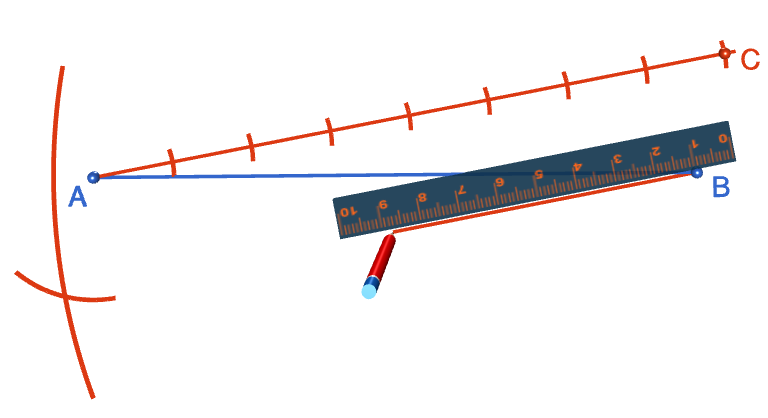Step 6: Using your straight edge, draw a line segment joining point $B$ to point $D$.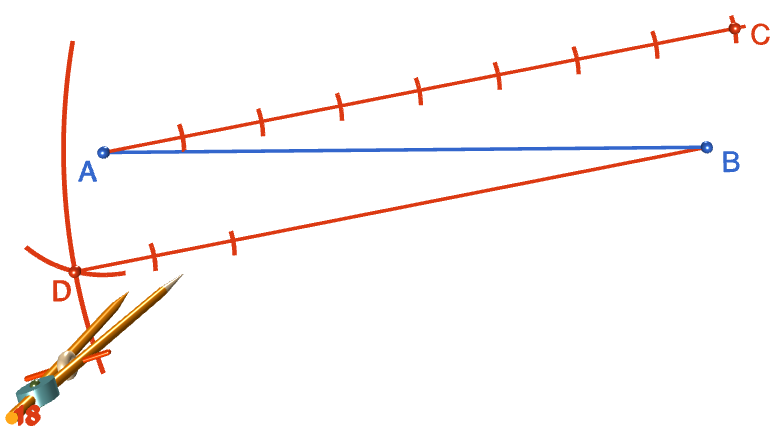Step 7: Open out your compasses to a radius that matches the distance between point $A$ and the intersection of the first arc drawn at step 2 with the upper line segment. Making sure your compasses are centred on point $D$, draw an arc across line $BD$. Step the compasses along line $BD$, drawing arcs as in step 2. You should draw a total of 7 arcs if you want to split your line segment into 8 pieces, 6 arcs if you want to split it into 7 pieces, and so on. As you draw each arc, make sure that your compasses are centred on the point of intersection of the preceding arc with line $BD$. The compasses are a bit off in the picture, but the arcs are drawn accurately.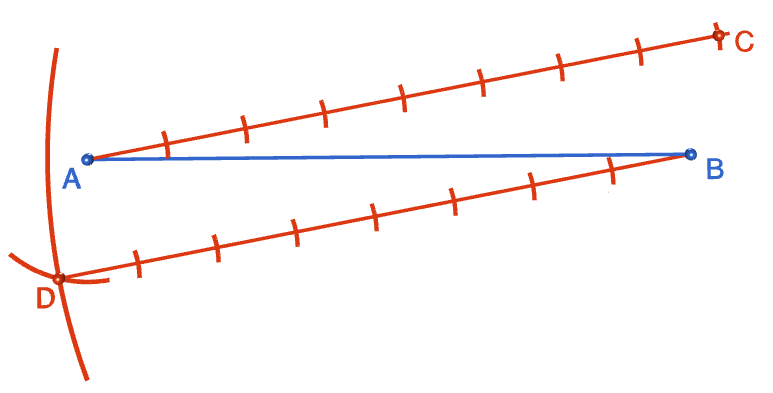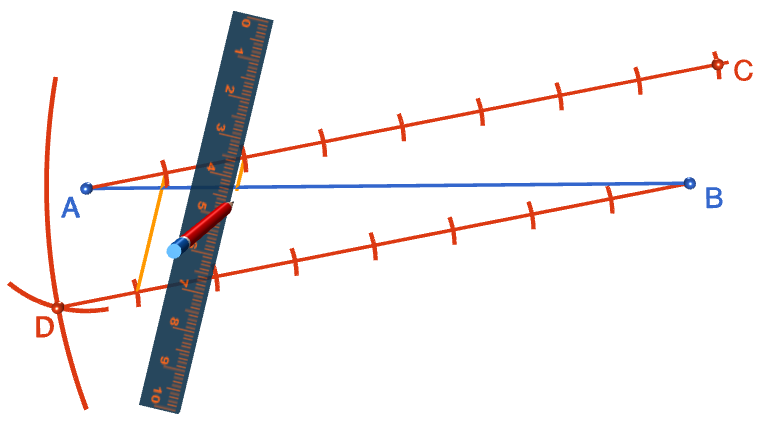Step 8: Using your straight edge, draw the lines between the corresponding points of the line segments $AC$ and $BD$. The result is a line segment that is divided into $N$ (8 in my case) equal parts.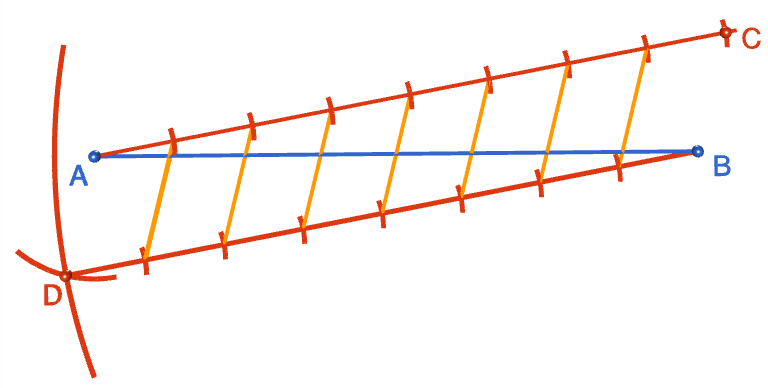Sit back and admire your work! You deserve a pat on the back.

### Description

This tutorial will expose you to Rulers and how to use them. We will also have a close look as set square and compass constructions. Your feedback is important to us, if you like any other topic covered under this tutorial, please do let us know.

### Audience

Year 10 or higher

### Learning Objectives

Ruler and compass constructions and more

Author: Subject Coach
You must be logged in as Student to ask a Question.